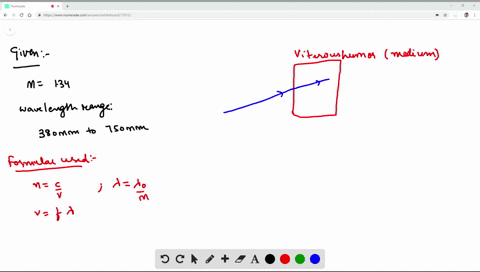Enroll in one of our FREE online STEM summer camps. Space is limited so join now!View Summer Courses### The vitreous humor, a transparent, gelatinous flu…

05:06Wesleyan University

Need more help? Fill out this quick form to get professional live tutoring.

Get live tutoring
Problem 1

Two plane mirrors intersect at right angles. A laser beam strikes the first of them
at a point 11.5 cm from their point of intersection, as shown in $\textbf{Fig. E33.1}$. For what angle of incidence at the first mirror will this ray strike the midpoint of the second mirror (which is 28.0 cm long) after reflecting from the first mirror?

$$39.4^{\circ}$$

## Discussion

You must be signed in to discuss.

## Video Transcript

this problem. We have two mirrors that are perpendicular to each other and light strikes this first mirror and bounces off back. And then it's done. The problem is asked for what? Angle off incident at the first mirror. Will the rail strike midpoint of the second mirror, which is 28 sending me to the law. So then we will find this angle. And from this angle, this is normal. Will find this angle this angle off incident. Hey, um, So we confined far this angle, which is saying that can be found Or let me call this fi. So let's call this fi. So this is Fi. So this is Fi. So this is after this is oh, fight Here is the fi So then this will be fine. So sorry about that mess. And, uh so this is fire. So Tang Fei will be equal to 14 centimeter because off the midpoint and then the 11 it strikes the from here to here From here to here that's 11 on from here to here. That's 14. So off 11.5, If we divide this and then sold for If I then the five you get here is 50.6 degrees, and then we confined angle off reflection from here. Just objecting. This, um, this are We can subtract a five from 90 degrees and then, uh, we get this angle 39.4, and then we know this angle of reflection should equal to angle off incident for this case, off playing mirrors. And so the angle off incident is denying 0.4 degrees. End off the problem. Thank you.